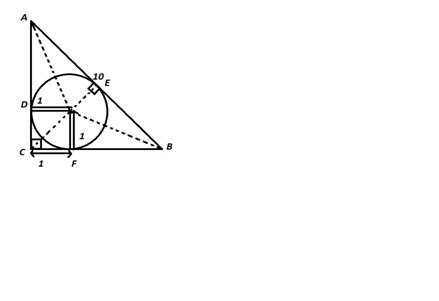#### Thank you for registering.

One of our academic counsellors will contact you within 1 working day.

Click to Chat

1800-1023-196

+91-120-4616500

CART 0

• 0
MY CART (5)

Use Coupon: CART20 and get 20% off on all online Study Material

ITEM
DETAILS
MRP
DISCOUNT
FINAL PRICE
Total Price: Rs.

There are no items in this cart.
Continue Shopping

# A circle is incribed in a right angled triangle of hpotenuse 10 units.if the radius of incribbed circle is 1 unit what is he perimenter of triangle

10 years ago

consider the figure

(AB)^2=(AC)^2+(BC)^2 ----------- Pythagoras

consider AD = BF = x

and CF=DC=1 because radius is equal to 1

100=(x+1)^2+(x+1)^2

which implies x^2+2x-49

solving through quad equation we get x=-1+5(2)^1/2 and -1-5(2)^1/2

perimeter = AB+BC+AC = 10+x+1+x+1 = 2x+12 = 2(x+6)

possible value of x = -1+5(2)^1/2

subtituting tha valu of x gives u perimeter = 2(5+5(2)^1/2) = 10+10(2)^1/2

(2)^1/2 means root 2

10(2)^1/2 means 10 root 210 years ago

a2+b2=c2 (c=10)-------- (1)

from Δle ICF ; IC=√2

→ CE= 1+√2

In ΔleCEB ; cos(C/2) = CE/a where C=90o

→ a= 2+√2

→ put a= 2+√2 and c=10 in (1) and b = 2√ (26-√2)

hence perimeter = a+b+c = 12+√2+2√(26-√2)